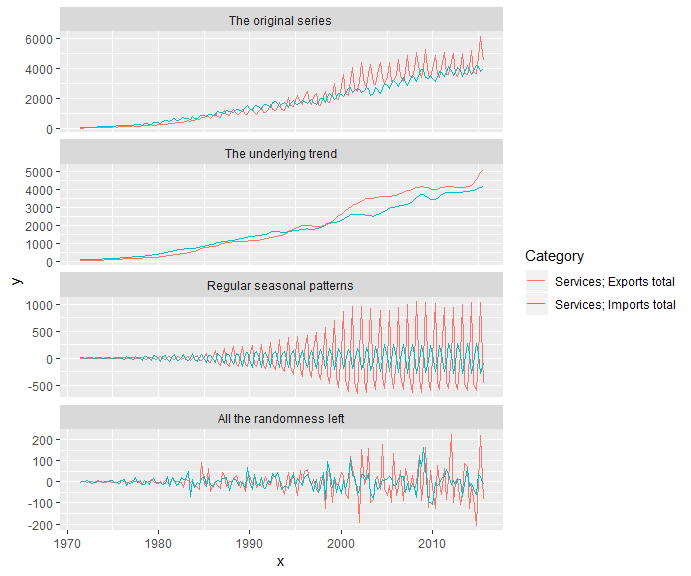ggseas - seasonal decomposition on the fly

Seasonal decomposition on the fly

The ggseas R package aims to help exploratory analysis of time series by making it easy to do seasonal adjustment and decomposition on the fly in the ggplot2 universe. It provides two main sets of functionality:

• a collection of ggplot2 stats oriented to time-series, allowing indexing, rolling averages and seasonal decomposition to be added as straight-forward statistical transforms with familiar geoms like geom_line and geom_point.
• the ggsdc() function which provides decomposition similar to that from decompose, stl or the X13-SEATS-ARIMA world via the seasonal package, except in ggplot2 terms so you can use familiar themes, titles, control your geoms and scales, etc. - and have multiple time series decomposed on a single graphic.

Using stats for simple time series graphic composition

ggseas provides five stats that have geom_line as their default geom and will easily integrate into a time series exploration:

• stat_index
• stat_decomp
• stat_rollapplyr
• stat_stl
• stat_seas

Like everything in the ggplot2 universe, these need your data to be in a data.frame (or its modern cousin the tibble). Much time series analysis in R uses ts and mts objects rather than data.frames so a first step to use ggplot2 for time series is to convert your data into a data.frame. The very simple tsdf function is provided to make this easier (there must be someone who’s done this earlier and better than me, if so let me know). It extracts the information on time from a ts or mts object and makes it a column, with time series data columns to the right of it:

library(ggseas)
ap_df <- tsdf(AirPassengers)
x   y
1 1949.000 112
2 1949.083 118
3 1949.167 132
4 1949.250 129
5 1949.333 121
6 1949.417 135

This means we can draw our time series in the usual ggplot2 way:

ggplot(ap_df, aes(x = x, y = y)) +
geom_line(colour = "grey75")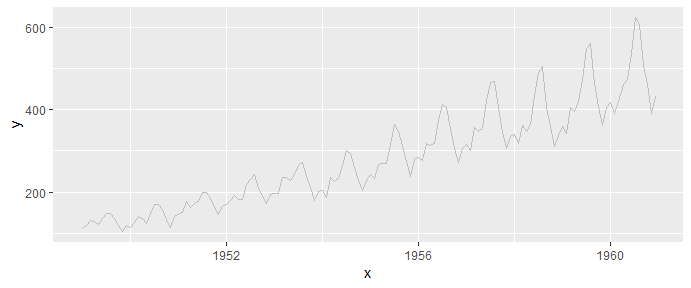Convert your data to an index with stat_index()

One thing we often want to do with time series data is turn it into an index with some arbitrarily chosen point as a reference, often set to equal 100. The stat_index() stat does this for you. In the case below, the average value of the first 10 points of the data is chosen as the reference to equal the default of 100.

ggplot(ap_df, aes(x = x, y = y)) +
stat_index(index.ref = 1:10)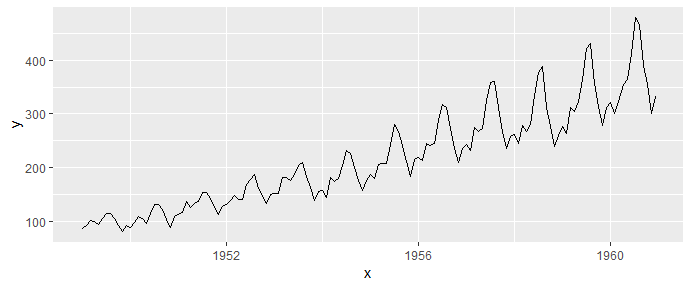You can control all that. For example, you might want the 120th point of the data to be equal to 1000

ggplot(ap_df, aes(x = x, y = y)) +
stat_index(index.ref = 120, index.basis = 1000)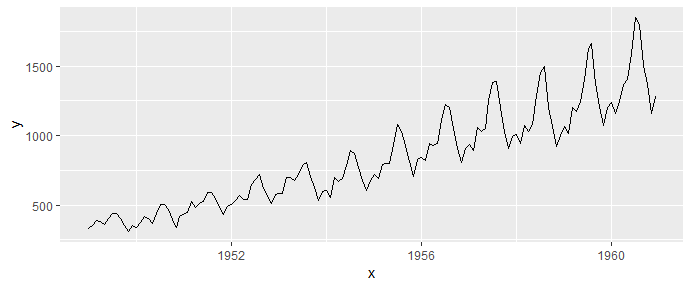Indexing also turns up later as an option in most of the other functions in ggseas.

Make rolling averages easily with stat_rollapplyr()

stat_rollapplyr() makes it easy to add a rolling function to your data, defaulting to the mean:

ggplot(ap_df, aes(x = x, y = y)) +
geom_line(colour = "grey75") +
stat_rollapplyr(width = 12, align = "center") +
labs(x = "", y = "Number of US Air Passengers\n(rolling average and original)")
Warning: Removed 11 rows containing missing values (geom_path).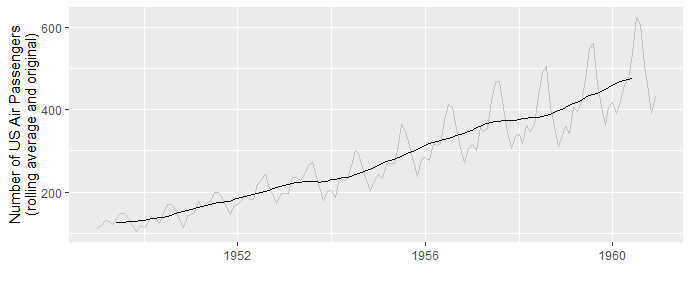It’s not very likely you’ll want anything but a line for a graphic like this, but in case you do, you can over-write the default geom. And of course all the usual ggplot2 polishing facilities are available:

ggplot(ap_df, aes(x = x, y = y)) +
geom_line(colour = "grey75") +
stat_rollapplyr(width = 12, align = "center", geom = "point",
size = 0.5, colour = "steelblue") +
labs(x = "", y = "Number of US Air Passengers\n(rolling average and original)")
Warning: Removed 11 rows containing missing values (geom_point).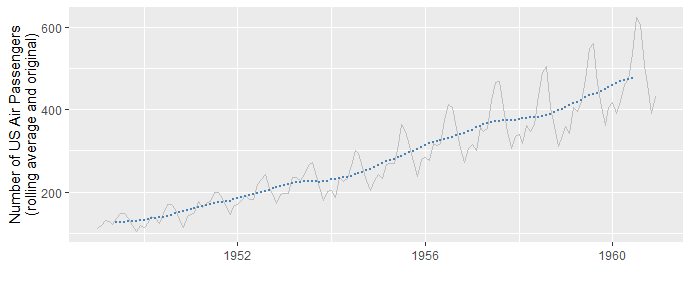Three stats will do seasonal adjustment for you, but I recommend only two of them: stat_stl() and stat_seas(). In fact, if stat_seas works it will generally be better. Amongst other things it does automatic detection of outliers and level shifts, best transformation to make, and adjustments for Easter and number of trading days. It’s driven by Christopher Sax’s seasonal R package, which is the best interface on the planet to the X13-SEATS-ARIMA time series analysis application from the US Census Department, which is the industry standard particularly for official statistics agencies doing seasonal adjustment.

X13 (via stat_seas) has limitations, such as a maximum number of observations, and only working with socio-economic style data (eg things measured in months, quarters and year rather than in milliseconds or eons). For these cases, stat_stl() provides a robust workhorse, a wrapper around stl() that ships with R in the stats package and implements the Clevelands’ seasonal-trend decomposition procedure based on a loess scatterplot smoother.

Here they both are in action:

ggplot(ap_df, aes(x = x, y = y)) +
geom_line(colour = "grey50") +
stat_seas(colour = "blue") +
stat_stl(s.window = 7, colour = "red")
Calculating starting date of 1949 from the data.
Calculating frequency of 12 from the data.
Calculating frequency of 12 from the data.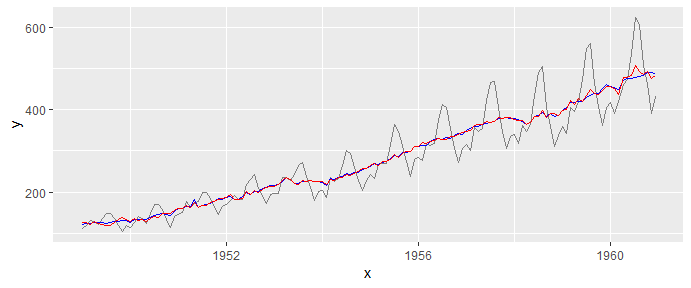Check out the helpfiles for more options. For example, you can convert the data to an index first, or pass more advanced arguments to the underlying time series programs.

One of the more interesting implications of bringing seasonal adjustment into the ggplot2 universe is the ability to slice and dice the data by aesthetic mappings (usually to colour) by faceting. This all works seamlessly in a way that will be familiar to ggplot2 users eg

ggplot(ldeaths_df, aes(x = YearMon, y = deaths, colour = sex)) +
geom_point() +
facet_wrap(~sex) +
stat_seas() +
ggtitle("Seasonally adjusted lung deaths in the UK")
Calculating starting date of 1974 from the data.
Calculating frequency of 12 from the data.
Calculating starting date of 1974 from the data.
Calculating frequency of 12 from the data.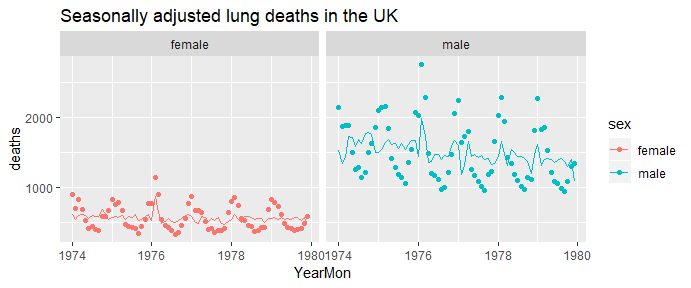ggsdc for quick decomposition into trend, seasonal and random components

The final key functionality from the ggseas package is the ability to do decomposition into trend, seasonal and random components, within the ggplot paradigm for polishing, aesthetic mappings, etc. Unlike the stat_seas() and its cousins which are stats that fit into the usual ggplot() + ... +... pipeline, ggsdc() is a replacement for the ggplot() function that normally starts that pipeline. ggsdc() has to seize control of the faceting operations in the pipeline and a few other things so it can’t be added just as a geom (at least not to this author’s understanding).

ggsdc() provides access to classic decomposition (additive or multiplicative) from decompose(), loess-based via stl(), or X13-SEATS-ARIMA via seasonal(). Again, I recommend one of the latter two as the more powerful and flexible approaches to seasonality, much better at handling slowly changing seasonality over time.

Here’s how it works at its simplest:

ggsdc(ap_df, aes(x = x, y = y), method = "seas") + geom_line()
Calculating starting date of 1949 from the data.
Calculating frequency of 12 from the data.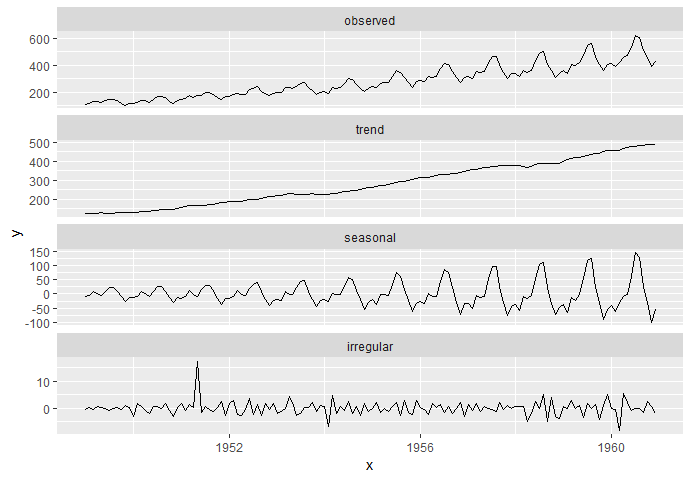Note that you still need to add a geom (usually geom_line()) to tell ggplot2 how to render the chart

Here’s a slightly more complex version with a multivariate series:

serv <- subset(nzbop, Account == "Current account" &
Category %in% c("Services; Exports total", "Services; Imports total"))
ggsdc(serv, aes(x = TimePeriod, y = Value, colour = Category),
method = "stl", s.window = 7, frequency = 4,
facet.titles = c("The original series", "The underlying trend",
"Regular seasonal patterns", "All the randomness left")) +
geom_line()